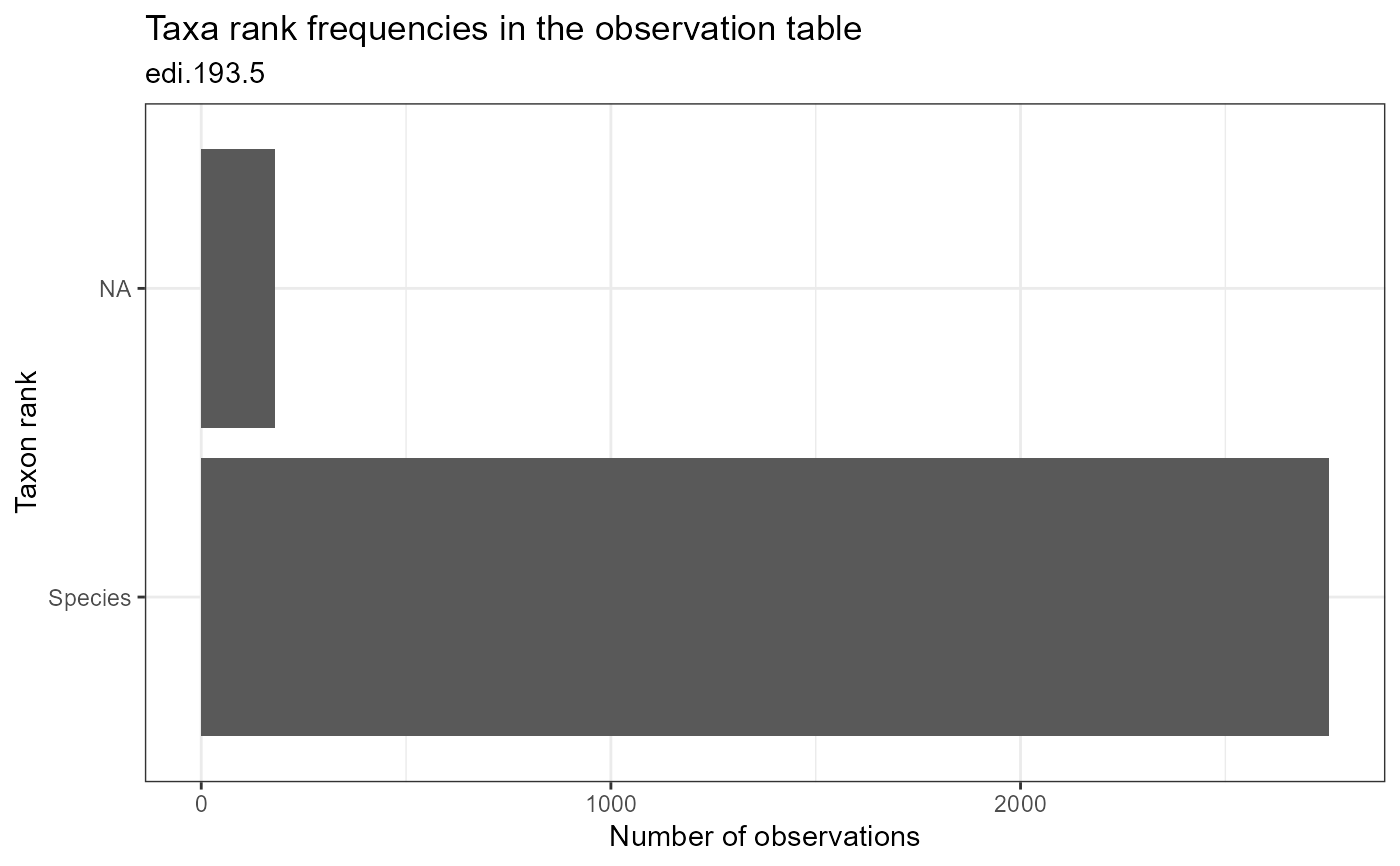Plot the number of observations that use each taxonomic rank in the dataset.

plot_taxa_rank(
data,
id = NA_character_,
facet_var = NA_character_,
facet_scales = "free",
alpha = 1
)

Arguments

data (list or tbl_df, tbl, data.frame) The dataset object returned by read_data(), a named list of tables containing the observation and taxon tables, or a flat table containing columns of the observation and taxon tables. (character) Identifier of dataset to be used in plot subtitles. Is automatically assigned when data is a dataset object containing the id field, or is a table containing the package_id column. (character) Name of column to use for faceting. Must be a column of the observation or taxon table. (character) Should scales be free ("free", default value), fixed ("fixed"), or free in one dimension ("free_x", "free_y")? (numeric) Alpha-transparency scale of data points. Useful when many data points overlap. Allowed values are between 0 and 1, where 1 is 100% opaque. Default is 1.

Value

(gg, ggplot) A gg, ggplot object if assigned to a variable, otherwise a plot to your active graphics device

Details

The data parameter accepts a range of input types but ultimately requires the 13 columns of the combined observation and taxon tables.

Examples

if (FALSE) { # Read a dataset of interest dataset <- read_data( id = "neon.ecocomdp.20120.001.001", site= c('COMO','LECO'), startdate = "2017-06", enddate = "2019-09", check.size = FALSE) # Plot the dataset plot_taxa_rank(dataset) # Plot with facet by location plot_taxa_rank(dataset, facet_var = "location_id") # Flatten the dataset, manipulate, then plot dataset %>% flatten_data() %>% dplyr::filter(lubridate::as_date(datetime) > "2003-07-01") %>% dplyr::filter(grepl("COMO",location_id)) %>% plot_taxa_rank() } # Plot the example dataset plot_taxa_rank(ants_L1)